Anzeige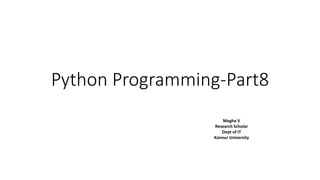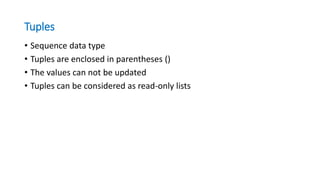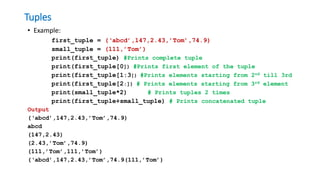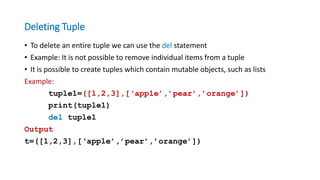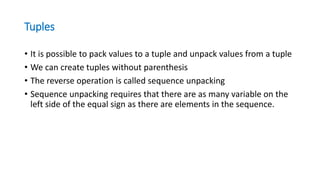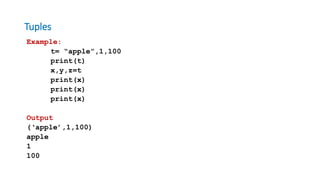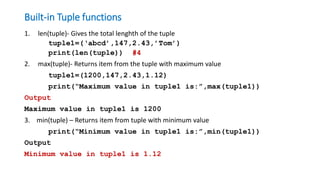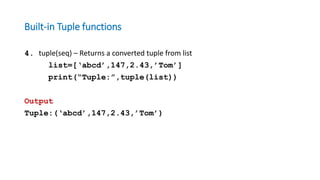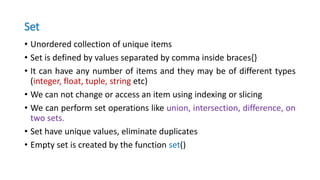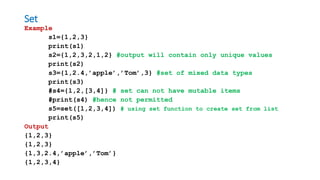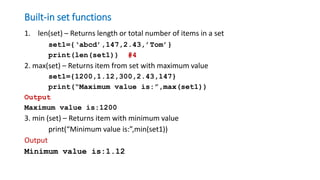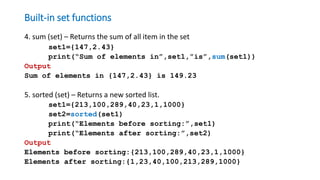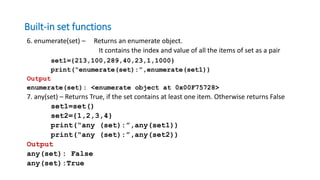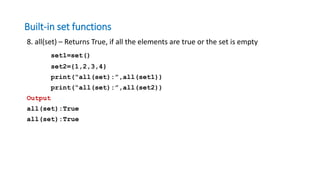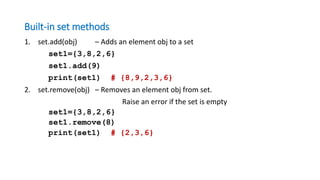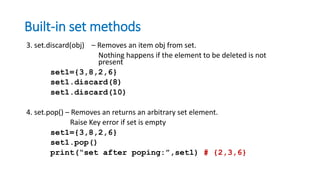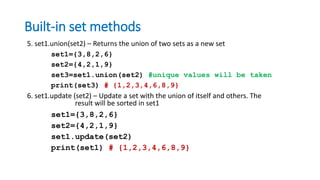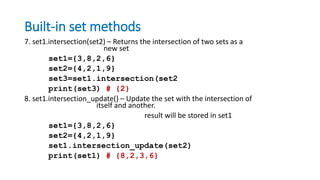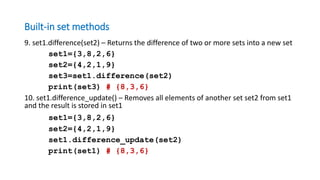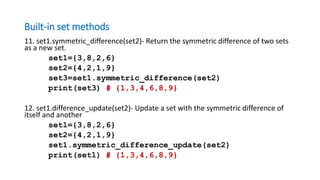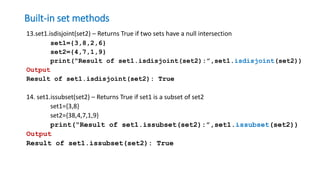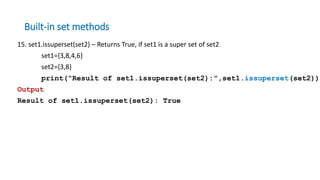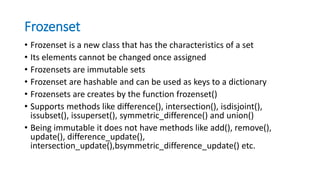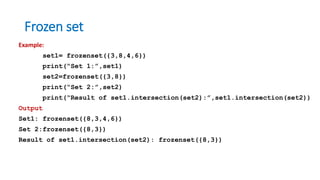1 von 24
Anzeige

### Python programming -Tuple and Set Data type

1. Python Programming-Part8 Megha V Research Scholar Dept of IT Kannur University
2. Tuples • Sequence data type • Tuples are enclosed in parentheses () • The values can not be updated • Tuples can be considered as read-only lists
3. Tuples • Example: first_tuple = (‘abcd’,147,2.43,’Tom’,74.9) small_tuple = (111,’Tom’) print(first_tuple) #Prints complete tuple print(first_tuple) #Prints first element of the tuple print(first_tuple[1:3]) #Prints elements starting from 2nd till 3rd print(first_tuple[2:]) # Prints elements starting from 3rd element print(small_tuple*2) # Prints tuples 2 times print(first_tuple+small_tuple) # Prints concatenated tuple Output (‘abcd’,147,2.43,’Tom’,74.9) abcd (147,2.43) (2.43,’Tom’,74.9) (111,’Tom’,111,’Tom’) (‘abcd’,147,2.43,’Tom’,74.9(111,’Tom’)
4. Deleting Tuple • To delete an entire tuple we can use the del statement • Example: It is not possible to remove individual items from a tuple • It is possible to create tuples which contain mutable objects, such as lists Example: tuple1=([1,2,3],[‘apple’,’pear’,’orange’]) print(tuple1) del tuple1 Output t=([1,2,3],[‘apple’,’pear’,’orange’])
5. Tuples • It is possible to pack values to a tuple and unpack values from a tuple • We can create tuples without parenthesis • The reverse operation is called sequence unpacking • Sequence unpacking requires that there are as many variable on the left side of the equal sign as there are elements in the sequence.
6. Tuples Example: t= “apple”,1,100 print(t) x,y,z=t print(x) print(x) print(x) Output (‘apple’,1,100) apple 1 100
7. Built-in Tuple functions 1. len(tuple)- Gives the total lenghth of the tuple tuple1=(‘abcd’,147,2.43,’Tom’) print(len(tuple)) #4 2. max(tuple)- Returns item from the tuple with maximum value tuple1=(1200,147,2.43,1.12) print(“Maximum value in tuple1 is:”,max(tuple1)) Output Maximum value in tuple1 is 1200 3. min(tuple) – Returns item from tuple with minimum value print(“Minimum value in tuple1 is:”,min(tuple1)) Output Minimum value in tuple1 is 1.12
8. Built-in Tuple functions 4. tuple(seq) – Returns a converted tuple from list list=[‘abcd’,147,2.43,’Tom’] print(“Tuple:”,tuple(list)) Output Tuple:(‘abcd’,147,2.43,’Tom’)
9. Set • Unordered collection of unique items • Set is defined by values separated by comma inside braces{} • It can have any number of items and they may be of different types (integer, float, tuple, string etc) • We can not change or access an item using indexing or slicing • We can perform set operations like union, intersection, difference, on two sets. • Set have unique values, eliminate duplicates • Empty set is created by the function set()
10. Set Example s1={1,2,3} print(s1) s2={1,2,3,2,1,2} #output will contain only unique values print(s2) s3={1,2.4,’apple’,’Tom’,3} #set of mixed data types print(s3) #s4={1,2,[3,4]} # set can not have mutable items #print(s4) #hence not permitted s5=set([1,2,3,4]) # using set function to create set from list print(s5) Output {1,2,3} {1,2,3} {1,3,2.4,’apple’,’Tom’} {1,2,3,4}
11. Built-in set functions 1. len(set) – Returns length or total number of items in a set set1={‘abcd’,147,2.43,’Tom’} print(len(set1)) #4 2. max(set) – Returns item from set with maximum value set1={1200,1.12,300,2.43,147} print(“Maximum value is:”,max(set1)) Output Maximum value is:1200 3. min (set) – Returns item with minimum value print(“Minimum value is:”,min(set1)) Output Minimum value is:1.12
12. Built-in set functions 4. sum (set) – Returns the sum of all item in the set set1={147,2.43} print(“Sum of elements in”,set1,”is”,sum(set1)) Output Sum of elements in {147,2.43} is 149.23 5. sorted (set) – Returns a new sorted list. set1={213,100,289,40,23,1,1000} set2=sorted(set1) print(“Elements before sorting:”,set1) print(“Elements after sorting:”,set2) Output Elements before sorting:{213,100,289,40,23,1,1000} Elements after sorting:{1,23,40,100,213,289,1000}
13. Built-in set functions 6. enumerate(set) – Returns an enumerate object. It contains the index and value of all the items of set as a pair set1={213,100,289,40,23,1,1000} print(“enumerate(set):”,enumerate(set1)) Output enumerate(set): <enumerate object at 0x00F75728> 7. any(set) – Returns True, if the set contains at least one item. Otherwise returns False set1=set() set2={1,2,3,4} print(“any (set):”,any(set1)) print(“any (set):”,any(set2)) Output any(set): False any(set):True
14. Built-in set functions 8. all(set) – Returns True, if all the elements are true or the set is empty set1=set() set2={1,2,3,4} print(“all(set):”,all(set1)) print(“all(set):”,all(set2)) Output all(set):True all(set):True
15. Built-in set methods 1. set.add(obj) – Adds an element obj to a set set1={3,8,2,6} set1.add(9) print(set1) # {8,9,2,3,6} 2. set.remove(obj) – Removes an element obj from set. Raise an error if the set is empty set1={3,8,2,6} set1.remove(8) print(set1) # {2,3,6}
16. Built-in set methods 3. set.discard(obj) – Removes an item obj from set. Nothing happens if the element to be deleted is not present set1={3,8,2,6} set1.discard(8) set1.discard(10) 4. set.pop() – Removes an returns an arbitrary set element. Raise Key error if set is empty set1={3,8,2,6} set1.pop() print(“set after poping:”,set1) # {2,3,6}
17. Built-in set methods 5. set1.union(set2) – Returns the union of two sets as a new set set1={3,8,2,6} set2={4,2,1,9} set3=set1.union(set2) #unique values will be taken print(set3) # {1,2,3,4,6,8,9} 6. set1.update (set2) – Update a set with the union of itself and others. The result will be sorted in set1 set1={3,8,2,6} set2={4,2,1,9} set1.update(set2) print(set1) # {1,2,3,4,6,8,9}
18. Built-in set methods 7. set1.intersection(set2) – Returns the intersection of two sets as a new set set1={3,8,2,6} set2={4,2,1,9} set3=set1.intersection(set2 print(set3) # {2} 8. set1.intersection_update() – Update the set with the intersection of itself and another. result will be stored in set1 set1={3,8,2,6} set2={4,2,1,9} set1.intersection_update(set2) print(set1) # {8,2,3,6}
19. Built-in set methods 9. set1.difference(set2) – Returns the difference of two or more sets into a new set set1={3,8,2,6} set2={4,2,1,9} set3=set1.difference(set2) print(set3) # {8,3,6} 10. set1.difference_update() – Removes all elements of another set set2 from set1 and the result is stored in set1 set1={3,8,2,6} set2={4,2,1,9} set1.difference_update(set2) print(set1) # {8,3,6}
20. Built-in set methods 11. set1.symmetric_difference(set2)- Return the symmetric difference of two sets as a new set. set1={3,8,2,6} set2={4,2,1,9} set3=set1.symmetric_difference(set2) print(set3) # {1,3,4,6,8,9} 12. set1.difference_update(set2)- Update a set with the symmetric difference of itself and another set1={3,8,2,6} set2={4,2,1,9} set1.symmetric_difference_update(set2) print(set1) # {1,3,4,6,8,9}
21. Built-in set methods 13.set1.isdisjoint(set2) – Returns True if two sets have a null intersection set1={3,8,2,6} set2={4,7,1,9} print(“Result of set1.isdisjoint(set2):”,set1.isdisjoint(set2)) Output Result of set1.isdisjoint(set2): True 14. set1.issubset(set2) – Returns True if set1 is a subset of set2 set1={3,8} set2={38,4,7,1,9} print(“Result of set1.issubset(set2):”,set1.issubset(set2)) Output Result of set1.issubset(set2): True
22. Built-in set methods 15. set1.issuperset(set2) – Returns True, if set1 is a super set of set2 set1={3,8,4,6} set2={3,8} print(“Result of set1.issuperset(set2):”,set1.issuperset(set2)) Output Result of set1.issuperset(set2): True
23. Frozenset • Frozenset is a new class that has the characteristics of a set • Its elements cannot be changed once assigned • Frozensets are immutable sets • Frozenset are hashable and can be used as keys to a dictionary • Frozensets are creates by the function frozenset() • Supports methods like difference(), intersection(), isdisjoint(), issubset(), issuperset(), symmetric_difference() and union() • Being immutable it does not have methods like add(), remove(), update(), difference_update(), intersection_update(),bsymmetric_difference_update() etc.
24. Frozen set Example: set1= frozenset({3,8,4,6}) print(“Set 1:”,set1) set2=frozenset({3,8}) print(“Set 2:”,set2) print(“Result of set1.intersection(set2):”,set1.intersection(set2)) Output Set1: frozenset({8,3,4,6}) Set 2:frozenset({8,3}) Result of set1.intersection(set2): frozenset({8,3})
Anzeige